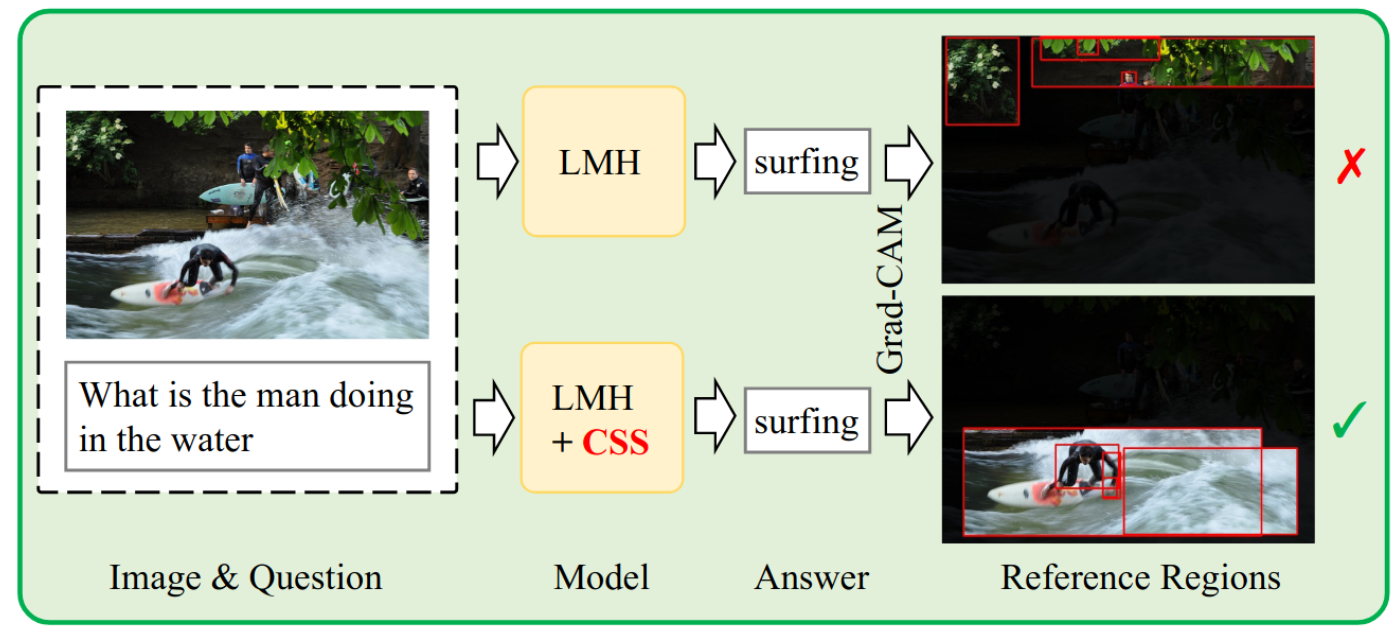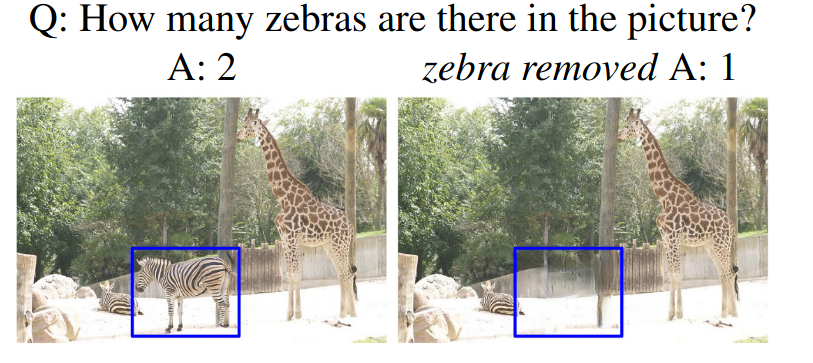We present various methods designed to reduce biases learned by deep learning models. Most methods will focus on the Visual Answering task, but might be applicable to many other tasks.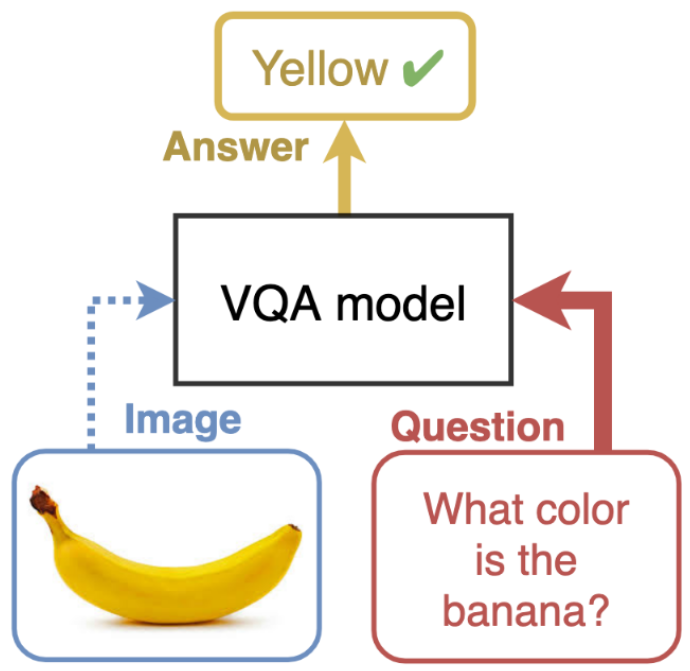### Intro on Visual Question Answering

Visual Question Answering task consists in answering a question about an image. For instance, in the previous figure, the question is “What color is the banana ?”, the correct answer is thus yellow.

Visual Question Answering has been shown to contain lot of unwanted biases, that enables models to reach high accuracy, but fail to answer for the right reasons. For instance, in VQA datasets, most bananas are yellow, and a model would fail to answer if it saw a green banana.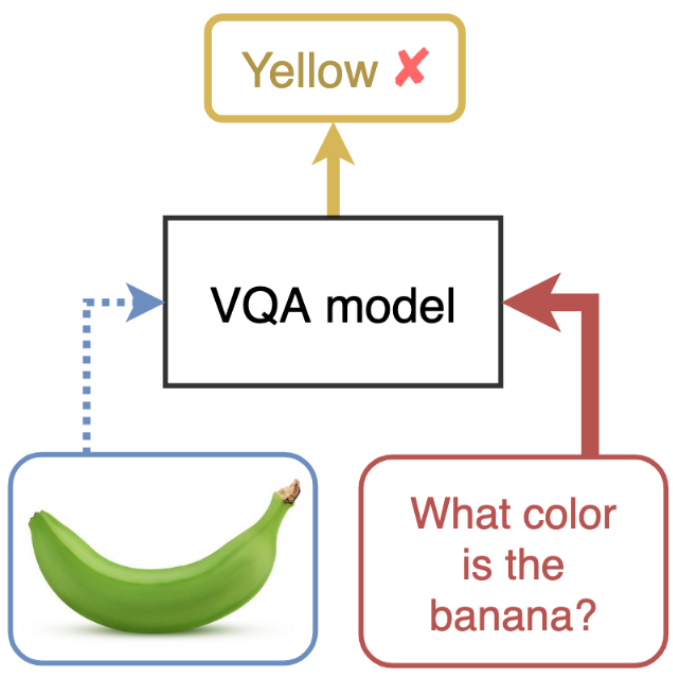### Evaluation is challenging

It is difficult to evaluate correctly a VQA model. Testing sets usually follow the same distribution as the training sets, thus they contain the same biases or shortcuts. The main solution that was proposed is to create out-of-distribution datasets, with the assumption that a biased model would fail to answer in rare cases, because it learned superficial correlations, while a correct model would manage to answer even in contexts that are different from its training set.

Even with this out-of-distribution setup, correct evaluation is challenging, as it does not match the usual assumptions made in machine learning. The validations set used for hyper parameter optimization and early-stopping cannot be out-of-distribution, or there is a risk of overfitting the out-of-distribution testing set biases, instead of learning a robust behaviour.

Another challenge is the dataset creation : It can be hard to gather OOD data that are not too different from the trainig set (that could still be answered from data in the training set). Usually, OOD datasets are obtained by reorganizing or removing examples from training and testing sets.

For Visual Question Anwering, the most used dataste is VQA-CP, presented in the paper Don’t Just Assume; Look and Answer: Overcoming Priors for Visual Question Answering by Agrawal et.al. It consists in changing the answer distribution conditionned on the type of question. For example, when the question starts with “what sport”, the most common answer will be “tennis” in the training set, and “skiing” in the testing set. This dataset will penalize models which rely too much on the question without looking at the image to answer.

A leaderboard of all papers targeting VQA-CP is available here : https://github.com/cdancette/vqa-cp-leaderboard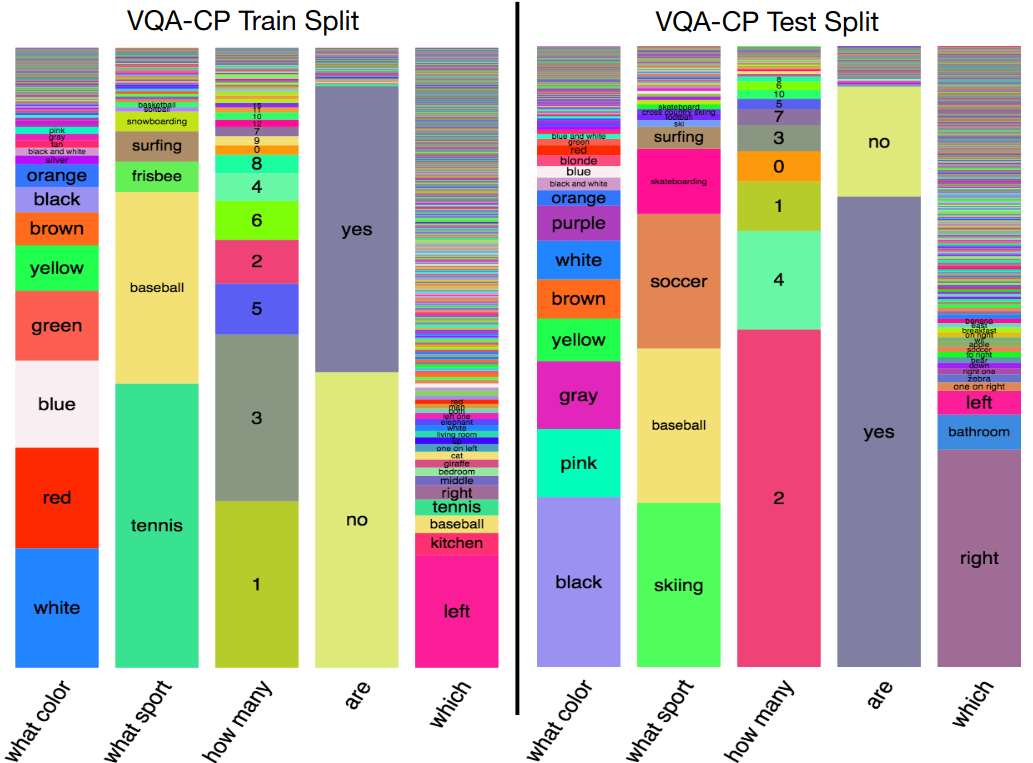We will now go over several bias-reduction methods: Simple baselines, Architectural priors, adversarial methods, model ensembling and data augmentation. Each of those methods may be adaptable to other tasks that suffer from biases.

### Baseline method: Reweighting / Sampling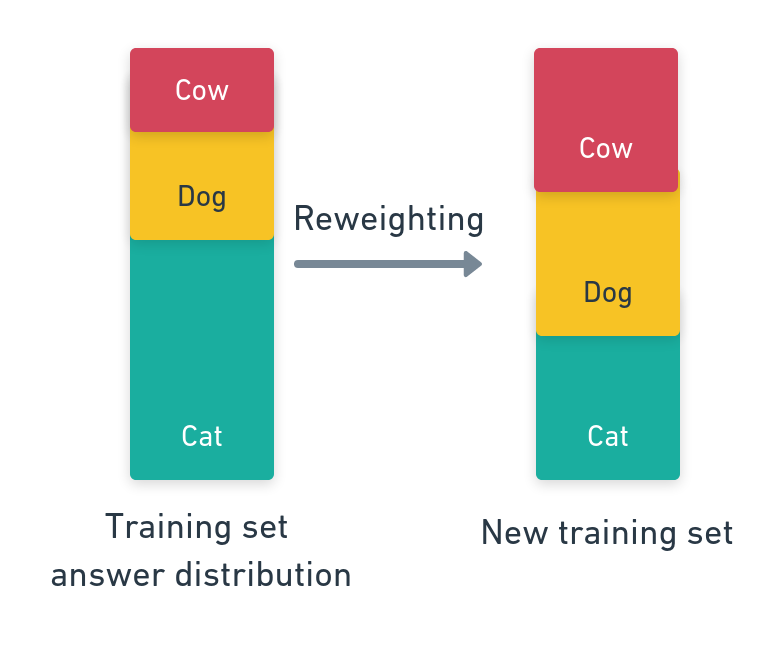The first straightforward method that can help to reduce biases in certain cases is reweighting your dataset. The idea is to over-sample difficult or rare examples, and under-sample common and easy examples. This will help for problems that suffer from an imbalance in labels / input concepts. For example, in Visual Question Answering, there often is an imbalance in the answer distribution. Reweighting can help

If we have an example $$X = (v, q, a) \in (I, Q, A)$$ where $$I$$ is the image set, $$Q$$ is the question set and $$A$$ is the answer set, we can sample our example X with the uniform probability for answers $$p(a) = 1/\|A\|$$

We are here introducing a new bias to reverse the main bias: that all answers are equally important, and they must be seen an equal number of times. There is a risk of overfitting on rare examples that will be oversampled.

### Architectural priors

When encountering biases, or spurious patterns that enables the model to answer correctly without any understanding, a natural solution is to incorporate domain knowledge into the model’s architecture. This will force the model to make its decision in a specific way, making some shortcuts impossible to capture.

##### GVQA

An example of this approach is the Grounded Visual Question Answering model (GVQA), presented in the Don’t Just Assume; Look and Answer: Overcoming Priors for Visual Question Answering paper from Agrawal et. al. It introduced VQA-Changing-Prior (VQA-CP) dataset, and the GVQA model.The idea developed in this article is that the question should only direct the decision towards a group of answers, and the image should help to choose among those answers. For example, for the question What color is the car ?, then the model will predict, from the question, a cluster of possible answers (here, all colors). Then, the vision model will predict all possible answers it can see in the image (it will be the name of the object present in the scene, the color of objects, or other attributes). Both predictions will then be aggregated in order to choose the final answer.

Here, we see that the question does not influence directly the decision, it just predicts a cluster of answers (we simplified a bit, as there is a specific branch for yes/no questions).

##### VGQE

Another example of an architectural prior is proposed in the paper Reducing Language Biases in Visual Question Answering with Visually-Grounded Question Encoder, by KV et. al, published in ECCV 2020. Here, they integrate the vision modality directly into the word embeddings, based on a similarity measure, before the RNN text encoding. This forces the model to take into account the vision modality very early in the decision. Interestingly, it does not hurt performances on the original VQA val set, contrarily to most bias-reduction methods.

Adversarial losses can be used to reduce biases coming from a known factor. For example, in Visual Question Answering, question is a factor of bias, which means that models rely too much on the question, and not often on the image, because the question alone is often sufficient to answer.

The main idea of the adversarial loss is to force the model to be wrong when given only the question.

An example of such an approach is the paper Overcoming Language Priors in Visual Question Answering with Adversarial Regularization, which was published at NeurIPS 2018.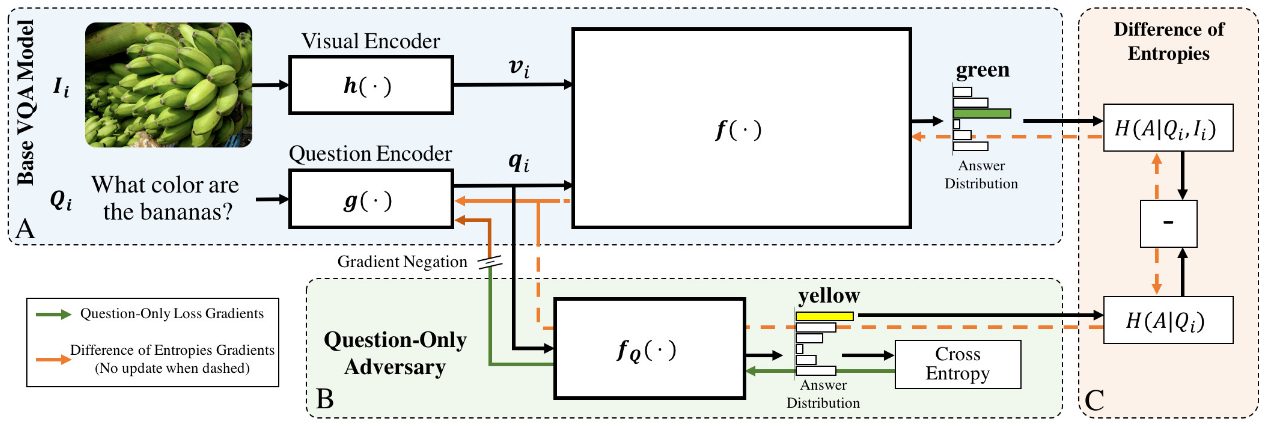In this paper, they introduce a question-only classifier, that shares the question encoder with the main VQA model. The question-only classifier is trained as an adversarial classifier: Its gradients are reversed before flowing into the question encoder. This means that the question-encoder must learn a question representation that makes it harder for the question-only model to answer the question alone, while still allowing the main VQA model to answer. The ultimate goal is to reduce the question-related biases.

Additionaly, they introduce the Difference of Entropy loss: it forces the entropy from the questoin-only model to be different from the entropy of the main VQA model. This means that the additional image information should make the model more certain about its answer than the question-only model. Note that this loss only updates the parametrs of the question encoder.

The main limitation of this approach is that it only affects the question encoder, and not the vision-encoder, or the fusion block, where biases can still be captured.

### Ensembling-based regularization

Ensembling-based regularization uses the idea of training two models as an ensembling: our main model, and a weak biased model. The biased model might be pretrained or not, depending on the approach. This will allow the weak model to capture the shallow or spurious patterns, and let the main model focus on the more difficult examples, making it more robust.

The weak model can be a partial model (looking at only a fraction of the input), or a shallow model that has a much lower number of parameters compared to our original model.

Contrarily to the previous approach, this will have an effect on the full VQA model, instead of the question-encoder only.

An example of this approach for VQA is RUBi: Reducing Unimodal Biases in Visual Question Answering (this work was done by Remi Cadene and myself).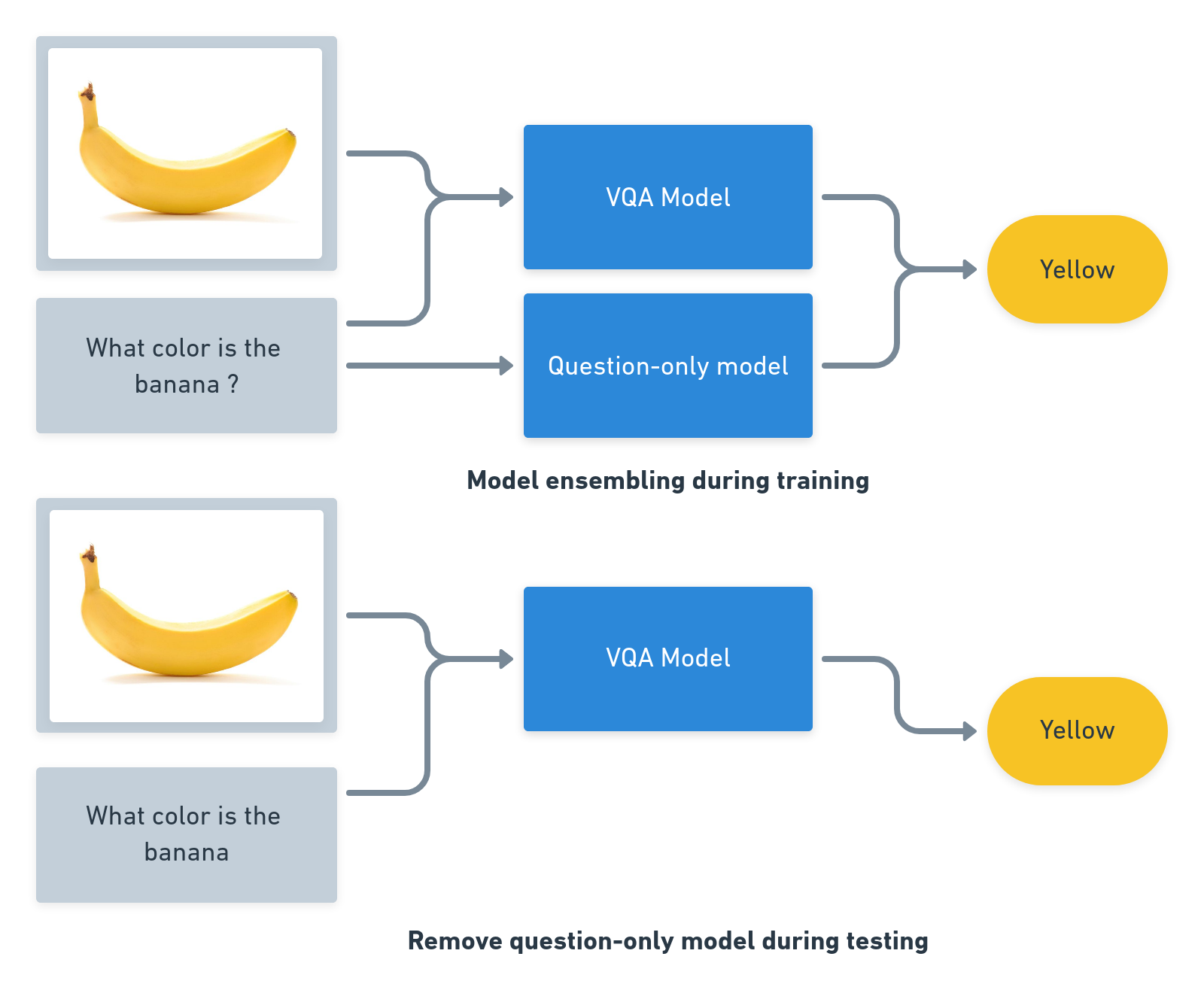In the RUBi approach, the weak model is a question-only model. This choice was made because VQA is known to display biases towards the question modality. So our ensembling will enable the model to learn behaviours that are less dependant on the question alone, letting the question-only model capturing question biases.

### Structuring the output loss

The main idea of this approach is to help the model to make sense of the output : Instead of telling it that it is just right or wrong, we will give additional information: how wrong it it ?

For example, a model answering the question “What is the color of the car ?”, given a green car, is more wrong if it answers “football” than “grey”. Or for a counting question, if the answer is 2, it’s better to answer 3 than 10.

For counting, the correct structure for the loss would be a regression: using L2, you get more correction if you’re very far from the correct answer, and very little if you’re close. For example, the paper Overcoming Statistical Shortcuts for Open-ended Visual Counting targets this problem, and shows that using the regression, instead of classification, helps to reduce biases.

For the general VQA problem, it’s more challenging, as answers are extremely varied. The paper Estimating semantic structure for the VQA answer space targets this issue. They first introduce a measure of similarity for VQA answers, which is based on co-occurences in the multiple answers for a single questions. Two answers are very similar if they were used as answers for the same question-image. Then, they introduce an additional loss, which penalizes more the model when the answer is far from the ground truth answer.

### Data-augmentation based regularization

Data augmentation can be a powerful mechanism to reduce biases in models. It can be to create an invariance to a specific transformation that might otherwise induce biases, or to make the model understand what is the important features in the data.

For instance, in vision models, data augmentation can be used to force models to be invariant to color changes, rotations or symetries, or noise, and might help against biases or adversarial attacks.

##### Counterfactual-based data augmentation

For visual question answering, one approach that was used is to create counterfactual examples: minor modifications of the image of the question that will change the answer. The intuition is that will help the model figure out which part of the input is really important, and will remove some biases. The goal is now to figure out how to find important image regions and words, and how to modify them. Another question is how to use the additional examples: simply adding them to the training set, or using aditional losses to give information to the model.